# General decay for a system of nonlinear viscoelastic wave equations with weak damping

## Abstract

In this paper, we are concerned with a system of nonlinear viscoelastic wave equations with initial and Dirichlet boundary conditions in ${\mathbb{R}}^{n}$ ($n=1,2,3$). Under suitable assumptions, we establish a general decay result by multiplier techniques, which extends some existing results for a single equation to the case of a coupled system.

MSC:35L05, 35L55, 35L70.

## 1 Introduction

In this paper, we are concerned with a coupled system of nonlinear viscoelastic wave equations with weak damping

(1.1)

where $\mathrm{\Omega }\subseteq {\mathbb{R}}^{n}$ ($n=1,2,3$) is a bounded domain with smooth boundary Ω, u and v represent the transverse displacements of waves. The functions ${g}_{1}$ and ${g}_{2}$ denote the kernel of a memory, ${f}_{1}\left(u,v\right)$ and ${f}_{2}\left(u,v\right)$ are the nonlinearities.

In recent years, many mathematicians have paid their attention to the energy decay and dynamic systems of the nonlinear wave equations, hyperbolic systems and viscoelastic equations.

Firstly, we recall some results concerning single viscoelastic wave equation. Kafini and Tatar  considered the following Cauchy problem:

$\left\{\begin{array}{c}{u}_{tt}-\mathrm{\Delta }u+{\int }_{0}^{t}g\left(t-s\right)\mathrm{\Delta }u\left(x,s\right)\phantom{\rule{0.2em}{0ex}}ds=0,\phantom{\rule{1em}{0ex}}x\in {\mathbb{R}}^{n},t>0,\hfill \\ u\left(x,0\right)={u}_{0}\left(x\right),\phantom{\rule{2em}{0ex}}{u}_{t}\left(x,0\right)={u}_{1}\left(x\right),\phantom{\rule{1em}{0ex}}x\in {\mathbb{R}}^{n}.\hfill \end{array}$
(1.2)

They established the polynomial decay of the first-order energy of solutions for compactly supported initial data and for a not necessarily decreasing relaxation function. Later Tatar  studied the problem (1.2) with the Dirichlet boundary condition and showed that the decay of solutions was an arbitrary decay not necessarily at exponential or polynomial rate. Cavalcanti et al.  studied the following equation with Dirichlet boundary condition:

${|{u}_{t}|}^{\rho }{u}_{tt}-\mathrm{\Delta }u-\mathrm{\Delta }{u}_{tt}+g\ast \mathrm{\Delta }u-\gamma \mathrm{\Delta }{u}_{t}=0.$

The authors established a global existence result for $\gamma \ge 0$ and an exponential decay of energy for $\gamma >0$. They studied the interaction within the ${|{u}_{t}|}^{\rho }{u}_{tt}$ and the memory term $g\ast \mathrm{\Delta }u$. Later on, several other results were published based on . For more results on a single viscoelastic equation, we can refer to .

For a coupled system, Agre and Rammaha  investigated the following system:

where $\mathrm{\Omega }\subseteq {\mathbb{R}}^{n}$ ($n=1,2,3$) is a bounded domain with smooth boundary. They considered the following assumptions on ${f}_{i}$ ($i=1,2$):

(A1) Let

$F\left(u,v\right)=a{|u+v|}^{p+1}+2b{|uv|}^{\frac{p+1}{2}},\phantom{\rule{2em}{0ex}}{f}_{1}\left(u,v\right)=\frac{\partial F}{\partial u},\phantom{\rule{2em}{0ex}}{f}_{2}\left(u,v\right)=\frac{\partial F}{\partial v}$

with $a,b>0$, $p\ge 3$ if $n=1,2$ and $p=3$ if $n=3$; $m,r\ge 1$.

(A2) There exist two positive constants ${c}_{0}$, ${c}_{1}$ such that for all $u,v\in {\mathbb{R}}^{2}$, $F\left(u,v\right)$ satisfies

${c}_{0}\left({|u|}^{p+1}+{|v|}^{p+1}\right)\le F\left(u,v\right)\le {c}_{1}\left({|u|}^{p+1}+{|v|}^{p+1}\right).$

Under the assumptions (A1)-(A2), they established the global existence of weak solutions and the global existence of small weak solutions with initial and Dirichlet boundary conditions. Moreover, they also obtained the blow up of weak solutions. Mustafa  studied the following system:

$\left\{\begin{array}{c}{u}_{tt}-\mathrm{\Delta }u+{\int }_{0}^{t}{g}_{1}\left(t-\tau \right)\mathrm{\Delta }u\left(\tau \right)\phantom{\rule{0.2em}{0ex}}d\tau +{f}_{1}\left(u,v\right)=0,\hfill \\ {v}_{tt}-\mathrm{\Delta }v+{\int }_{0}^{t}{g}_{2}\left(t-\tau \right)\mathrm{\Delta }v\left(\tau \right)\phantom{\rule{0.2em}{0ex}}d\tau +{f}_{2}\left(u,v\right)=0,\hfill \end{array}$
(1.3)

in $\mathrm{\Omega }×\left(0,+\mathrm{\infty }\right)$ with initial and Dirichlet boundary conditions, proved the existence and uniqueness to the system by using the classical Faedo-Galerkin method and established a stability result by multiplier techniques. But the author considered the following different assumptions on ${f}_{i}$ ($i=1,2$) from (A1)-(A2):

(${\mathrm{A}}_{1}^{\prime }$) ${f}_{i}:{\mathbb{R}}^{2}\to \mathbb{R}$ ($i=1,2$) are ${C}^{1}$ functions and there exists a function F such that

${f}_{1}\left(x,y\right)=\frac{\partial F}{\partial x},\phantom{\rule{2em}{0ex}}{f}_{2}\left(x,y\right)=\frac{\partial F}{\partial y},\phantom{\rule{1em}{0ex}}F\ge 0,x{f}_{1}\left(x,y\right)+y{f}_{2}\left(x,y\right)\ge F\left(x,y\right),$

(${\mathrm{A}}_{2}^{\prime }$)

$|\frac{\partial {f}_{i}}{\partial x}\left(x,y\right)|+|\frac{\partial {f}_{i}}{\partial y}\left(x,y\right)|\le d\left(1+{|x|}^{{\beta }_{i1}-1}+{|y|}^{{\beta }_{i2}-1}\right),$

for all $\left(x,y\right)\in {\mathbb{R}}^{2}$, where the constant $d>0$ and ${\beta }_{ij}\ge 1$, $\left(n-2\right){\beta }_{ij}\le n$ for $i,j=1,2$.

Han and Wang  considered the following coupled nonlinear viscoelastic wave equations with weak damping:

(1.4)

where $\mathrm{\Omega }\subseteq {\mathbb{R}}^{n}$ is a bounded domain with smooth boundary Ω. Under the assumptions (A1)-(A2) on ${f}_{i}$ ($i=1,2$), the initial data and the parameters in the equations, they established the local existence, global existence uniqueness and finite time blow up properties. When the weak damping terms ${|{u}_{t}|}^{m-1}{u}_{t}$, ${|{v}_{t}|}^{r-1}{v}_{t}$ were replaced by the strong damping terms $-\mathrm{\Delta }{u}_{t}$, $-\mathrm{\Delta }{v}_{t}$, Liang and Gao  showed that under certain assumption on initial data in the stable set, the decay rate of the solution energy is exponential when they take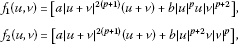$a,b>0$ and $p>-1$ if $n=1,2$, $-1 if $n=3$. Moreover, they obtained that the solutions with positive initial energy blow up in a finite time for certain initial data in the unstable set. For more results on coupled viscoelastic equations, we can refer to .

If we take $m=r=1$ in (1.4), the system will be transformed into (1.1). To the best of our knowledge, there is no result on general energy decay for the viscoelastic problem (1.1). Motivated by [16, 17], in this paper, we shall establish the general energy decay for the problem (1.1) by multiplier techniques, which extends some existing results for a single equation to the case of a coupled system. The rest of our paper is organized as follows. In Section 2, we give some preparations for our consideration and our main result. The statement and the proof of our main result will be given in Section 3.

For the reader’s convenience, we denote the norm and the scalar product in ${L}^{2}\left(\mathrm{\Omega }\right)$ by $\parallel \cdot \parallel$ and $\left(\cdot ,\cdot \right)$, respectively. ${C}_{1}$ denotes a general constant, which may be different in different estimates.

## 2 Preliminaries and main result

To state our main result, in addition to (A1)-(A2), we need the following assumption.

(A3) ${g}_{i}:{\mathbb{R}}^{+}\to {\mathbb{R}}^{+}$, $i=1,2$, are differentiable functions such that

${g}_{i}\left(0\right)>0,\phantom{\rule{1em}{0ex}}1-{\int }_{0}^{+\mathrm{\infty }}{g}_{i}\left(s\right)\phantom{\rule{0.2em}{0ex}}ds={l}_{i}>0,$

and there exist nonincreasing functions ${\xi }_{1},{\xi }_{2}:{\mathbb{R}}^{+}\to {\mathbb{R}}^{+}$ satisfying

${g}_{i}^{\prime }\left(t\right)\le -{\xi }_{i}\left(t\right){g}_{i}\left(t\right),\phantom{\rule{1em}{0ex}}t\ge 0.$

Now, we define the energy functional

$\begin{array}{rcl}E\left(t\right)& =& \frac{1}{2}{\int }_{\mathrm{\Omega }}\left({u}_{t}^{2}+\left(1-{\int }_{0}^{t}{g}_{1}\left(s\right)\phantom{\rule{0.2em}{0ex}}ds\right){|\mathrm{\nabla }u|}^{2}\right)\phantom{\rule{0.2em}{0ex}}dx\\ +\frac{1}{2}\left({g}_{1}\circ \mathrm{\nabla }u\right)\left(t\right)+\frac{1}{2}\left({g}_{2}\circ \mathrm{\nabla }v\right)\left(t\right)\\ +\frac{1}{2}{\int }_{\mathrm{\Omega }}\left({v}_{t}^{2}+\left(1-{\int }_{0}^{t}{g}_{2}\left(s\right)\phantom{\rule{0.2em}{0ex}}ds\right){|\mathrm{\nabla }v|}^{2}\right)\phantom{\rule{0.2em}{0ex}}dx-{\int }_{\mathrm{\Omega }}F\left(u,v\right)\phantom{\rule{0.2em}{0ex}}dx\end{array}$
(2.1)

and the functional

$\begin{array}{rcl}D\left(t\right)& =& \left(1-{\int }_{0}^{t}{g}_{1}\left(s\right)\phantom{\rule{0.2em}{0ex}}ds\right){\parallel \mathrm{\nabla }u\left(t\right)\parallel }^{2}+\left(1-{\int }_{0}^{t}{g}_{2}\left(s\right)\phantom{\rule{0.2em}{0ex}}ds\right){\parallel \mathrm{\nabla }v\left(t\right)\parallel }^{2}\\ +2\left[\left({g}_{1}\circ \mathrm{\nabla }u\right)\left(t\right)+\left({g}_{2}\circ \mathrm{\nabla }v\right)\left(t\right)\right]-4{\int }_{\mathrm{\Omega }}F\left(u\left(t\right),v\left(t\right)\right)\phantom{\rule{0.2em}{0ex}}dx,\end{array}$
(2.2)

where

$\left(g\circ y\right)\left(t\right)={\int }_{0}^{t}g\left(t-s\right){\parallel y\left(t\right)-y\left(s\right)\parallel }^{2}\phantom{\rule{0.2em}{0ex}}ds.$

The existence of a global solution to the system (1.1) is established in  as follows.

Proposition 

Let (A1)-(A3) hold. Assume that $D\left(0\right)={\parallel \mathrm{\nabla }{u}_{0}\parallel }^{2}+{\parallel \mathrm{\nabla }{v}_{0}\parallel }^{2}-4{\int }_{\mathrm{\Omega }}F\left({u}_{0},{v}_{0}\right)\phantom{\rule{0.2em}{0ex}}dx>0$, $\frac{{2}^{p}{C}_{0}}{l}{\left(\frac{E\left(0\right)}{l}\right)}^{\frac{p-1}{2}}<1$ and that $\left({u}_{0},{u}_{1}\right)\in {H}_{0}^{1}\left(\mathrm{\Omega }\right)×{L}^{2}\left(\mathrm{\Omega }\right)$, $\left({v}_{0},{v}_{1}\right)\in {H}_{0}^{1}\left(\mathrm{\Omega }\right)×{L}^{2}\left(\mathrm{\Omega }\right)$, where ${C}_{0}$ is a computable constant and $l=min\left\{{l}_{1},{l}_{2}\right\}$. Then the problem (1.1) has a unique global solution $\left(u\left(t\right),v\left(t\right)\right)$ satisfying

$\left(u\left(t\right),{u}_{t}\left(t\right)\right)\in C\left({\mathbb{R}}^{+};{H}_{0}^{1}\left(\mathrm{\Omega }\right)×{L}^{2}\left(\mathrm{\Omega }\right)\right),\phantom{\rule{1em}{0ex}}\left(v\left(t\right),{v}_{t}\left(t\right)\right)\in C\left({\mathbb{R}}^{+};{H}_{0}^{1}\left(\mathrm{\Omega }\right)×{L}^{2}\left(\mathrm{\Omega }\right)\right).$

We are now ready to state our main result.

Theorem 2.1 Let (A1)-(A3) hold. Assume that $D\left(0\right)={\parallel \mathrm{\nabla }{u}_{0}\parallel }^{2}+{\parallel \mathrm{\nabla }{v}_{0}\parallel }^{2}-4{\int }_{\mathrm{\Omega }}F\left({u}_{0},{v}_{0}\right)\phantom{\rule{0.2em}{0ex}}dx>0$, $\frac{{2}^{p}{C}_{0}}{l}{\left(\frac{E\left(0\right)}{l}\right)}^{\frac{p-1}{2}}<1$ and that $\left({u}_{0},{u}_{1}\right)\in {H}_{0}^{1}\left(\mathrm{\Omega }\right)×{L}^{2}\left(\mathrm{\Omega }\right)$, $\left({v}_{0},{v}_{1}\right)\in {H}_{0}^{1}\left(\mathrm{\Omega }\right)×{L}^{2}\left(\mathrm{\Omega }\right)$, where ${C}_{0}$ is a computable constant and $l=min\left\{{l}_{1},{l}_{2}\right\}$. Then there exist constants $C,\eta >0$ such that, for t large, the solution of (1.1) satisfies

$E\left(t\right)\le C{e}^{-\eta {\int }_{0}^{t}\xi \left(s\right)\phantom{\rule{0.2em}{0ex}}ds},$
(2.3)

where

$\xi \left(t\right)=min\left\{{\xi }_{1}\left(t\right),{\xi }_{2}\left(t\right)\right\},\phantom{\rule{1em}{0ex}}t\ge 0.$
(2.4)

## 3 Proof of Theorem 2.1

In this section, we carry out the proof of Theorem 2.1. Firstly, we will estimate several lemmas.

Lemma 3.1 Let $u\left(t\right)$, $v\left(t\right)$ be the solution of (1.1). Then the following energy estimate holds for any $t\ge 0$:

$\begin{array}{rcl}{E}^{\prime }\left(t\right)& =& -\left({\parallel {u}_{t}\parallel }^{2}+{\parallel {v}_{t}\parallel }^{2}\right)+\frac{1}{2}\left[\left({g}_{1}^{\prime }\circ \mathrm{\nabla }u\right)+\left({g}_{2}^{\prime }\circ \mathrm{\nabla }v\right)\right]\\ -\frac{1}{2}\left[{g}_{1}\left(t\right){\parallel \mathrm{\nabla }u\left(t\right)\parallel }^{2}+{g}_{2}\left(t\right){\parallel \mathrm{\nabla }v\left(t\right)\parallel }^{2}\right]\le 0.\end{array}$
(3.1)

Proof Multiplying the first equation of (1.1) by ${u}_{t}$ and the second equation by ${v}_{t}$, respectively, integrating the results over Ω, performing integration by parts and noting that ${F}_{t}\left(u,v\right)={f}_{1}\left(u,v\right){u}_{t}+{f}_{2}\left(u,v\right){v}_{t}$, we can easily get (3.1). The proof is complete. □

Lemma 3.2 Under the assumption (A3), the following hold: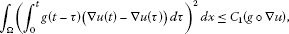(3.2)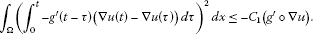(3.3)

Proof Using Hölder’s inequality, we get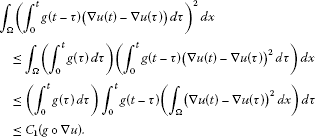On the other hand, we repeat the above proof with $-{g}^{\prime }$, instead of g, we can get (3.3). The proof is now complete. □

Lemma 3.3 Let (A1)-(A3) hold and $u\left(t\right)$, $v\left(t\right)$ be the solution of (1.1). Then the functional $I\left(t\right)$ defined by

$I\left(t\right):={\int }_{\mathrm{\Omega }}\left(u{u}_{t}+v{v}_{t}\right)\phantom{\rule{0.2em}{0ex}}dx$

satisfies

$\begin{array}{rcl}{I}^{\prime }\left(t\right)& \le & -\frac{{l}_{1}}{2}{\parallel \mathrm{\nabla }u\left(t\right)\parallel }^{2}-\frac{{l}_{2}}{2}{\parallel \mathrm{\nabla }v\left(t\right)\parallel }^{2}+\left(1+\frac{1}{4\delta }\right)\left({\parallel {u}_{t}\parallel }^{2}+{\parallel {v}_{t}\parallel }^{2}\right)\\ +\frac{{C}_{1}}{\delta }\left({g}_{1}\circ \mathrm{\nabla }u\right)+\frac{{C}_{1}}{\delta }\left({g}_{2}\circ \mathrm{\nabla }v\right)+{C}_{1}{\int }_{\mathrm{\Omega }}F\left(u,v\right)\phantom{\rule{0.2em}{0ex}}dx\end{array}$
(3.4)

for all $\delta >0$.

Proof By (1.1), a direct differentiation gives

$\begin{array}{rcl}{I}^{\prime }\left(t\right)& =& {\parallel {u}_{t}\parallel }^{2}-{\parallel \mathrm{\nabla }u\parallel }^{2}+{\int }_{\mathrm{\Omega }}\mathrm{\nabla }u{\int }_{0}^{t}{g}_{1}\left(t-\tau \right)\mathrm{\nabla }u\left(\tau \right)\phantom{\rule{0.2em}{0ex}}d\tau \phantom{\rule{0.2em}{0ex}}dx-{\int }_{\mathrm{\Omega }}{u}_{t}u\phantom{\rule{0.2em}{0ex}}dx+{\int }_{\mathrm{\Omega }}{f}_{1}u\phantom{\rule{0.2em}{0ex}}dx\\ +{\parallel {v}_{t}\parallel }^{2}-{\parallel \mathrm{\nabla }v\parallel }^{2}+{\int }_{\mathrm{\Omega }}\mathrm{\nabla }v{\int }_{0}^{t}{g}_{2}\left(t-\tau \right)\mathrm{\nabla }v\left(\tau \right)\phantom{\rule{0.2em}{0ex}}d\tau \phantom{\rule{0.2em}{0ex}}dx\\ -{\int }_{\mathrm{\Omega }}{v}_{t}v\phantom{\rule{0.2em}{0ex}}dx+{\int }_{\mathrm{\Omega }}{f}_{2}v\phantom{\rule{0.2em}{0ex}}dx.\end{array}$
(3.5)

From the assumptions (A1)-(A2), we derive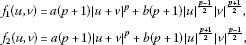and

$\begin{array}{rcl}{f}_{1}u+{f}_{2}v& =& a\left(p+1\right){|u+v|}^{p+1}+b\left(p+1\right){|uv|}^{\frac{p+1}{2}}\\ \le & {C}_{1}F\left(u,v\right).\end{array}$
(3.6)

By Young’s inequality and (3.2), we deduce for any $\delta >0$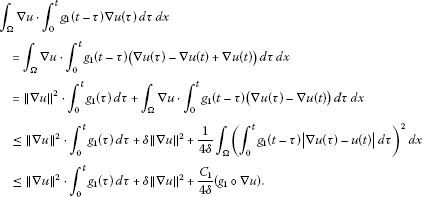(3.7)

Similarly, we have

$\begin{array}{rcl}{\int }_{\mathrm{\Omega }}\mathrm{\nabla }v\cdot {\int }_{0}^{t}{g}_{2}\left(t-\tau \right)\mathrm{\nabla }v\left(\tau \right)\phantom{\rule{0.2em}{0ex}}d\tau \phantom{\rule{0.2em}{0ex}}dx& \le & {\parallel \mathrm{\nabla }v\parallel }^{2}\cdot {\int }_{0}^{t}{g}_{2}\left(\tau \right)\phantom{\rule{0.2em}{0ex}}d\tau +\delta {\parallel \mathrm{\nabla }v\parallel }^{2}\\ +\frac{{C}_{1}}{4\delta }\left({g}_{2}\circ \mathrm{\nabla }v\right).\end{array}$
(3.8)

Using Young’s inequality and Poincaré’s inequality, we obtain for any $\delta >0$

${\int }_{\mathrm{\Omega }}u{u}_{t}\phantom{\rule{0.2em}{0ex}}dx\le \delta {\parallel u\parallel }^{2}+\frac{1}{4\delta }{\parallel {u}_{t}\parallel }^{2}\le \delta {\lambda }^{2}{\parallel \mathrm{\nabla }u\parallel }^{2}+\frac{1}{4\delta }{\parallel {u}_{t}\parallel }^{2},$
(3.9)

where λ is the first eigenvalue of −Δ with the Dirichlet boundary condition. Similarly,

${\int }_{\mathrm{\Omega }}v{v}_{t}\phantom{\rule{0.2em}{0ex}}dx\le \delta {\parallel v\parallel }^{2}+\frac{1}{4\delta }{\parallel {v}_{t}\parallel }^{2}\le \delta {\lambda }^{2}{\parallel \mathrm{\nabla }v\parallel }^{2}+\frac{1}{4\delta }{\parallel {v}_{t}\parallel }^{2},$

which together with (3.5)-(3.9) gives

$\begin{array}{rcl}{I}^{\prime }\left(t\right)& \le & -\left({l}_{1}-\delta -\delta {\lambda }^{2}\right){\parallel \mathrm{\nabla }u\parallel }^{2}-\left({l}_{2}-\delta -\delta {\lambda }^{2}\right){\parallel \mathrm{\nabla }v\parallel }^{2}+\left(1+\frac{1}{4\delta }\right)\left({\parallel {u}_{t}\parallel }^{2}+{\parallel {v}_{t}\parallel }^{2}\right)\\ +\frac{{C}_{1}}{4\delta }\left({g}_{1}\circ \mathrm{\nabla }u\right)+\frac{{C}_{1}}{4\delta }\left({g}_{2}\circ \mathrm{\nabla }v\right)+{C}_{1}{\int }_{\mathrm{\Omega }}F\left(u,v\right)\phantom{\rule{0.2em}{0ex}}dx.\end{array}$
(3.10)

Now, we choose $\delta >0$ so small that

${l}_{1}-\delta -\delta {\lambda }^{2}\ge \frac{{l}_{1}}{2},\phantom{\rule{2em}{0ex}}{l}_{2}-\delta -\delta {\lambda }^{2}\ge \frac{{l}_{2}}{2},$

which together with (3.10) gives (3.4). The proof is complete. □

Lemma 3.4 Let (A1)-(A3) hold and $u\left(t\right)$, $v\left(t\right)$ be the solution of (1.1). Then the functional $J\left(t\right)$ defined by

$J\left(t\right)={J}_{1}\left(t\right)+{J}_{2}\left(t\right),$

with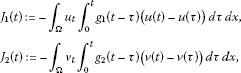satisfies

$\begin{array}{rcl}{J}^{\prime }\left(t\right)& \le & -\left({\int }_{0}^{t}{g}_{1}\left(\tau \right)-2\delta \right){\parallel {u}_{t}\parallel }^{2}+\delta {C}_{1}{\parallel \mathrm{\nabla }u\parallel }^{2}+\frac{{C}_{1}}{\delta }\left({g}_{1}\circ \mathrm{\nabla }u\right)-\frac{{C}_{1}}{\delta }\left({g}_{1}^{\prime }\circ \mathrm{\nabla }u\right)\\ -\left({\int }_{0}^{t}{g}_{2}\left(\tau \right)-2\delta \right){\parallel {v}_{t}\parallel }^{2}+\delta {C}_{1}{\parallel \mathrm{\nabla }v\parallel }^{2}+\frac{{C}_{1}}{\delta }\left({g}_{2}\circ \mathrm{\nabla }v\right)-\frac{{C}_{1}}{\delta }\left({g}_{2}^{\prime }\circ \mathrm{\nabla }v\right).\end{array}$
(3.11)

Proof A direct differentiation for ${J}_{1}\left(t\right)$ yields

$\begin{array}{rcl}{J}_{1}^{\prime }\left(t\right)& =& -{\int }_{\mathrm{\Omega }}{u}_{tt}\cdot {\int }_{0}^{t}{g}_{1}\left(t-\tau \right)\left(u\left(t\right)-u\left(\tau \right)\right)\phantom{\rule{0.2em}{0ex}}d\tau -{\int }_{\mathrm{\Omega }}{u}_{t}\cdot {\int }_{0}^{t}{g}_{1}^{\prime }\left(t-\tau \right)\left(u\left(t\right)-u\left(\tau \right)\right)\phantom{\rule{0.2em}{0ex}}d\tau \phantom{\rule{0.2em}{0ex}}dx\\ -\left({\int }_{0}^{t}{g}_{1}\left(\tau \right)\phantom{\rule{0.2em}{0ex}}d\tau \right){\int }_{\mathrm{\Omega }}{u}_{t}^{2}\phantom{\rule{0.2em}{0ex}}dx.\end{array}$
(3.12)

Using the first equation of (1.1) and integrating by parts, we obtain

$\begin{array}{rcl}{J}_{1}^{\prime }\left(t\right)& =& \left(1-{\int }_{0}^{t}{g}_{1}\left(\tau \right)\phantom{\rule{0.2em}{0ex}}d\tau \right){\int }_{\mathrm{\Omega }}\mathrm{\nabla }u\cdot {\int }_{0}^{t}{g}_{1}\left(t-\tau \right)\left(\mathrm{\nabla }u\left(t\right)-\mathrm{\nabla }u\left(\tau \right)\right)\phantom{\rule{0.2em}{0ex}}d\tau \phantom{\rule{0.2em}{0ex}}dx\\ +{\int }_{\mathrm{\Omega }}{\left({\int }_{0}^{t}{g}_{1}\left(t-\tau \right)|\mathrm{\nabla }u\left(t\right)-\mathrm{\nabla }u\left(\tau \right)|\phantom{\rule{0.2em}{0ex}}d\tau \right)}^{2}\phantom{\rule{0.2em}{0ex}}dx\\ +{\int }_{\mathrm{\Omega }}{u}_{t}\cdot {\int }_{0}^{t}{g}_{1}\left(t-\tau \right)\left(u\left(t\right)-u\left(\tau \right)\right)\phantom{\rule{0.2em}{0ex}}d\tau \phantom{\rule{0.2em}{0ex}}dx\\ -{\int }_{\mathrm{\Omega }}{f}_{1}\left(u,v\right){\int }_{0}^{t}{g}_{1}\left(t-\tau \right)\left(u\left(t\right)-u\left(\tau \right)\right)\phantom{\rule{0.2em}{0ex}}d\tau \phantom{\rule{0.2em}{0ex}}dx\\ -{\int }_{\mathrm{\Omega }}{u}_{t}\cdot {\int }_{0}^{t}{g}_{1}^{\prime }\left(t-\tau \right)\left(u\left(t\right)-u\left(\tau \right)\right)\phantom{\rule{0.2em}{0ex}}d\tau \phantom{\rule{0.2em}{0ex}}dx\\ -\left({\int }_{0}^{t}{g}_{1}\left(\tau \right)\phantom{\rule{0.2em}{0ex}}d\tau \right){\int }_{\mathrm{\Omega }}{u}_{t}^{2}\phantom{\rule{0.2em}{0ex}}dx.\end{array}$
(3.13)

From Young’s inequality, Poincaré’s inequality and Lemma 3.2, we derive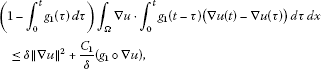(3.14)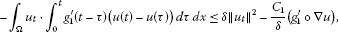(3.15)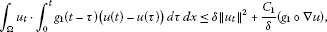(3.16)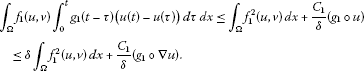(3.17)

Now, we estimate the first term on the right-hand side of (3.17). Using the assumptions (A1)-(A2) and Young’s inequality, we arrive at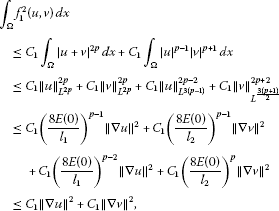(3.18)

where we used the embedding ${H}_{0}^{1}\left(\mathrm{\Omega }\right)↪{L}^{s}\left(\mathrm{\Omega }\right)$ for $2\le s\le 2n/\left(n-2\right)$ if $n=3$ or $s\ge 2$ if $n=1,2$ and the fact $\frac{1}{2}\left({\parallel {u}_{t}\parallel }^{2}+{\parallel {v}_{t}\parallel }^{2}\right)+\frac{1}{4}{l}_{1}{\parallel \mathrm{\nabla }u\parallel }^{2}+\frac{1}{4}{l}_{2}{\parallel \mathrm{\nabla }v\parallel }^{2}\le 2E\left(0\right)$ proved in Lemma 5.1 in . Combining (3.13)-(3.18), we get

$\begin{array}{rcl}{J}_{1}^{\prime }\left(t\right)& \le & -\left({\int }_{0}^{t}{g}_{1}\left(\tau \right)\phantom{\rule{0.2em}{0ex}}d\tau -2\delta \right){\parallel {u}_{t}\parallel }^{2}+\delta {C}_{1}{\parallel \mathrm{\nabla }u\parallel }^{2}+\delta {C}_{1}{\parallel \mathrm{\nabla }v\parallel }^{2}\\ +\frac{{C}_{1}}{\delta }\left({g}_{1}\circ \mathrm{\nabla }u\right)-\frac{{C}_{1}}{\delta }\left({g}_{1}^{\prime }\circ \mathrm{\nabla }u\right).\end{array}$
(3.19)

The same estimate to ${J}_{2}\left(t\right)$, we can derive

$\begin{array}{rcl}{J}_{2}^{\prime }\left(t\right)& \le & -\left({\int }_{0}^{t}{g}_{2}\left(\tau \right)\phantom{\rule{0.2em}{0ex}}d\tau -2\delta \right){\parallel {v}_{t}\parallel }^{2}+\delta {C}_{1}{\parallel \mathrm{\nabla }u\parallel }^{2}+\delta {C}_{1}{\parallel \mathrm{\nabla }v\parallel }^{2}\\ +\frac{{C}_{1}}{\delta }\left({g}_{2}\circ \mathrm{\nabla }v\right)-\frac{{C}_{1}}{\delta }\left({g}_{2}^{\prime }\circ \mathrm{\nabla }v\right),\end{array}$

which together with (3.19) gives (3.11). The proof is now complete. □

Proof of Theorem 2.1 For ${N}_{1},{N}_{2}>0$, we define the functional $\mathcal{K}$ by

$\mathcal{K}:={N}_{1}E\left(t\right)+{N}_{2}J\left(t\right)+I\left(t\right),$

and let

${g}_{0}=min\left\{{\int }_{0}^{{t}_{0}}{g}_{1}\left(s\right)\phantom{\rule{0.2em}{0ex}}ds,{\int }_{0}^{{t}_{0}}{g}_{2}\left(s\right)\phantom{\rule{0.2em}{0ex}}ds\right\}$

for some fixed ${t}_{0}>0$.

Using Lemma 3.1 and Lemmas 3.3-3.4, a direct differentiation gives

$\begin{array}{rcl}{\mathcal{K}}^{\prime }\left(t\right)& \le & -\left(\frac{l}{2}-{N}_{2}\delta {C}_{1}\right)\left({\parallel \mathrm{\nabla }u\parallel }^{2}+{\parallel \mathrm{\nabla }v\parallel }^{2}\right)+\left(\frac{{C}_{1}}{\delta }+{N}_{2}\frac{{C}_{1}}{\delta }\right)\left[\left({g}_{1}\circ \mathrm{\nabla }u\right)+\left({g}_{2}\circ \mathrm{\nabla }v\right)\right]\\ -\left({N}_{1}+{N}_{2}-2\delta -1-\frac{1}{4\delta }\right)\left({\parallel {u}_{t}\parallel }^{2}+{\parallel {v}_{t}\parallel }^{2}\right)+{C}_{1}{\int }_{\mathrm{\Omega }}F\left(u,v\right)\phantom{\rule{0.2em}{0ex}}dx\\ +\left(\frac{{N}_{1}}{2}-\frac{{N}_{2}{C}_{1}}{\delta }\right)\left[\left({g}_{1}^{\prime }\circ \mathrm{\nabla }u\right)+\left({g}_{2}^{\prime }\circ \mathrm{\nabla }v\right)\right],\end{array}$
(3.20)

where $l=min\left\{{l}_{1},{l}_{2}\right\}$.

Now, we choose $\delta =\frac{1}{4{C}_{1}{N}_{2}}$ and ${N}_{1}$, ${N}_{2}$ large enough so that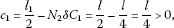(3.21)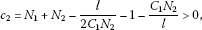(3.22)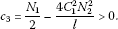(3.23)

Inserting (3.21)-(3.23) into (3.20), we have

$\begin{array}{rcl}{\mathcal{K}}^{\prime }\left(t\right)& \le & -{c}_{1}\left({\parallel \mathrm{\nabla }u\parallel }^{2}+{\parallel \mathrm{\nabla }v\parallel }^{2}\right)-{c}_{2}\left({\parallel {u}_{t}\parallel }^{2}+{\parallel {v}_{t}\parallel }^{2}\right)+{c}_{3}\left[\left({g}_{1}^{\prime }\circ \mathrm{\nabla }u\right)+\left({g}_{2}^{\prime }\circ \mathrm{\nabla }v\right)\right]\\ +\left(\frac{4{C}_{1}^{2}{N}_{2}}{l}+\frac{4{C}_{1}^{2}{N}_{2}}{l}\right)\left[\left({g}_{1}\circ \mathrm{\nabla }u\right)+\left({g}_{2}\circ \mathrm{\nabla }v\right)\right]+{C}_{1}{\int }_{\mathrm{\Omega }}F\left(u,v\right)\phantom{\rule{0.2em}{0ex}}dx.\end{array}$
(3.24)

Therefore, for two positive constants ω and C, we obtain

(3.25)

On the other hand, we choose ${N}_{1}$ even larger so that $\mathcal{K}\left(t\right)$ is equivalent to $E\left(t\right)$, i.e.,

$\mathcal{K}\left(t\right)\sim E\left(t\right).$
(3.26)

Multiplying (3.25) by $\xi \left(t\right)=min\left\{{\xi }_{1}\left(t\right),{\xi }_{2}\left(t\right)\right\}$ and using (A3), we get

(3.27)

By virtue of (A3) and $\xi \left(t\right)\le 0$, we have

(3.28)

Using (3.26), we can easily get

$\mathcal{L}\left(t\right):=\xi \left(t\right)\mathcal{K}\left(t\right)+CE\left(t\right)\sim E\left(t\right),$
(3.29)

which together with (3.28) yields, for some positive constant η,

(3.30)

Integrating (3.30) over $\left({t}_{0},t\right)$, we arrive at

$\begin{array}{rcl}\mathcal{L}\left(t\right)& \le & \mathcal{L}\left({t}_{0}\right){e}^{-\eta {\int }_{t}^{{t}_{0}}\xi \left(\tau \right)\phantom{\rule{0.2em}{0ex}}d\tau }\\ \le & C{e}^{-\eta {\int }_{t}^{{t}_{0}}\xi \left(\tau \right)\phantom{\rule{0.2em}{0ex}}d\tau },\end{array}$

which together with (3.29) and the boundedness of E and ξ yields (2.3). The proof is now complete. □

## References

1. Kafini M, Tatar N-e:A decay result to a viscoelastic in ${\mathbb{R}}^{n}$ with an oscillating kernel. J. Math. Phys. 2010., 51(7): Article ID 073506

2. Tatar N-e: Arbitrary decay in linear viscoelastic. J. Math. Phys. 2011., 52(1): Article ID 013502

3. Cavalcanti MM, Domingos Cavalcanti VN, Ferreira J: Existence and uniform decay for a non-linear viscoelastic equation with strong damping. Math. Methods Appl. Sci. 2001, 24: 1043-1053. 10.1002/mma.250

4. Cavalcanti MM, Domingos Cavalcanti VN, Prates Filho JS, Soriano JA: Existence and uniform decay rates for viscoelastic problems with non-linear boundary damping. Differ. Integral Equ. 2001, 14(1):85-116.

5. Messaoudi SA, Tatar N-e: Global existence and uniform stability of solutions for a quasilinear viscoelastic problem. Math. Methods Appl. Sci. 2007, 30: 665-680. 10.1002/mma.804

6. Messaoudi SA, Tatar N-e: Exponential and polynomial decay for a quasilinear viscoelastic problem. Math. Nachr. 2009, 282: 1443-1450. 10.1002/mana.200610800

7. Fabrizio M, Polidoro S: Asymptotic decay for some differential systems with fading memory. Appl. Anal. 2002, 81(6):1245-1264. 10.1080/0003681021000035588

8. Messaoudi SA: General decay of solutions of a viscoelastic equation. J. Math. Anal. Appl. 2008, 341: 1457-1467. 10.1016/j.jmaa.2007.11.048

9. Messaoudi SA: General decay of the solution energy in a viscoelastic equation with a nonlinear source. Nonlinear Anal. TMA 2008, 69: 2589-2598. 10.1016/j.na.2007.08.035

10. Messaoudi SA, Fareh A: General decay for a porous thermoelastic system with memory: the case of equal speeds. Nonlinear Anal. TMA 2011, 74: 6895-6906. 10.1016/j.na.2011.07.012

11. Messaoudi SA, Tatar N-e: Exponential and polynomial decay for a quasilinear viscoelastic equation. Nonlinear Anal. TMA 2007, 68: 785-793.

12. Tatar N-e: Exponential decay for a viscoelastic problem with a singular problem. Z. Angew. Math. Phys. 2009, 60(4):640-650. 10.1007/s00033-008-8030-1

13. Mediden M, Tatar N-e: Asymptotic behavior for a viscoelastic problem with not necessarily decreasing kernel. Appl. Math. Comput. 2005, 167(2):1221-1235. 10.1016/j.amc.2004.08.035

14. Liu W: General decay rate estimate for a viscoelastic equation with weakly nonlinear time-dependent dissipation and source terms. J. Math. Phys. 2009., 50(11): Article ID 113506

15. Agre K, Rammaha MA: System of nonlinear wave equations with damping and source terms. Differ. Integral Equ. 2006, 19: 1235-1270.

16. Mustafa MI: Well posedness and asymptotic behavior of a coupled system of nonlinear viscoelastic equations. Nonlinear Anal.: Real World Appl. 2012, 13: 452-463. 10.1016/j.nonrwa.2011.08.002

17. Han X, Wang M: Global existence and blow up of solutions for a system of nonlinear viscoelastic wave equations with damping and source. Nonlinear Anal. TMA 2009, 71: 5427-5450. 10.1016/j.na.2009.04.031

18. Liang F, Gao H: Exponential energy decay and blow up of solutions for a system of nonlinear viscoelastic wave equations with strong damping. Bound. Value Probl. 2011. doi:10.1186/1687-2770-2011-22

19. Liu W: Uniform decay of solutions for a quasilinear system of viscoelastic equations. Nonlinear Anal. TMA 2009, 71: 2257-2267. 10.1016/j.na.2009.01.060

20. Medeiros LA, Miranda MM: Weak solutions for a system of nonlinear Klein-Gordon equations. Ann. Mat. Pura Appl. 1987, 146: 173-183.

21. Zhang J: On the standing wave in coupled nonlinear Klein-Gordon equations. Math. Methods Appl. Sci. 2003, 26: 11-25. 10.1002/mma.340

## Acknowledgements

Baowei Feng was supported by the Doctoral Innovational Fund of Donghua University with contract number BC201138, and Yuming Qin was supported by NNSF of China with contract numbers 11031003 and 11271066 and the grant of Shanghai Education Commission (No. 13ZZ048).

## Author information

Authors

### Corresponding author

Correspondence to Baowei Feng.

### Competing interests

The authors declare that they have no competing interests.

### Authors’ contributions

The paper is a joint work of all authors who contributed equally to the final version of the paper. All authors read and approved the final manuscript.

## Rights and permissions

Reprints and Permissions

Feng, B., Qin, Y. & Zhang, M. General decay for a system of nonlinear viscoelastic wave equations with weak damping. Bound Value Probl 2012, 146 (2012). https://doi.org/10.1186/1687-2770-2012-146